Courses

# NCERT Exemplar - Statistics Class 10 Notes | EduRev

## Class 10 : NCERT Exemplar - Statistics Class 10 Notes | EduRev

The document NCERT Exemplar - Statistics Class 10 Notes | EduRev is a part of the Class 10 Course Mathematics (Maths) Class 10.
All you need of Class 10 at this link: Class 10

Q.1. Find the mean marks of students for the following distribution: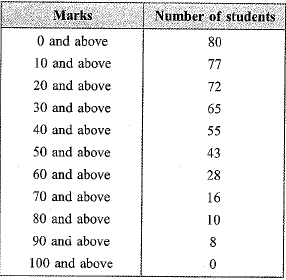Ans.
By step deviation method
Let a = 55 and h = 10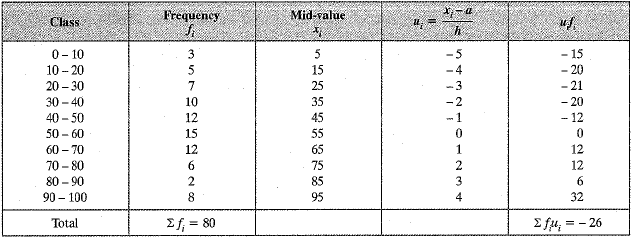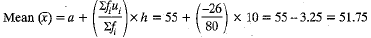Q.2. Determine the mean of the following distribution: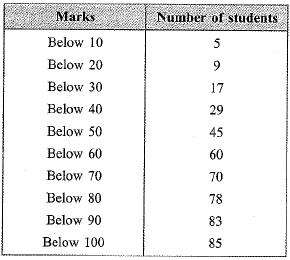Ans.
a = 45, h = 10.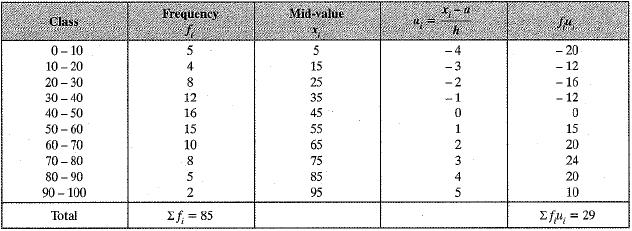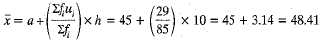Q.3. The following table shows the cumulative frequency distribution of marks of 800 students in an examination: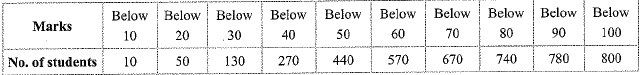Construct a frequency distribution table for the data above.
Ans.
Frequency distribution table: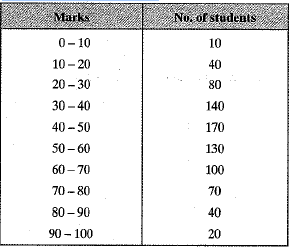Offer running on EduRev: Apply code STAYHOME200 to get INR 200 off on our premium plan EduRev Infinity!

## Mathematics (Maths) Class 10

51 videos|346 docs|103 tests

,

,

,

,

,

,

,

,

,

,

,

,

,

,

,

,

,

,

,

,

,

;